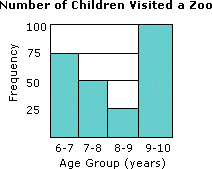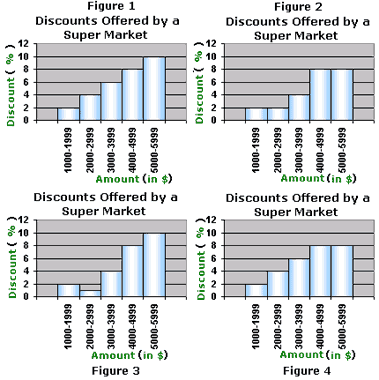Definition Of Histogram

A histogram is a bar graph that shows how frequently data occur within certain ranges or intervals. The height of each bar gives the frequency in the respective interval.

Examples of HistogramSolved Example on Histogram

Ques: The discounts offered by a super market are as shown in the table. Which of these histograms is the correct representation of the data?A. Figure 1
B. Figure 2
C. Figure 3
D. Figure 4